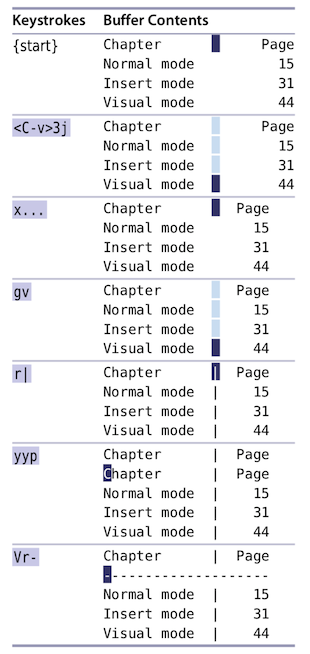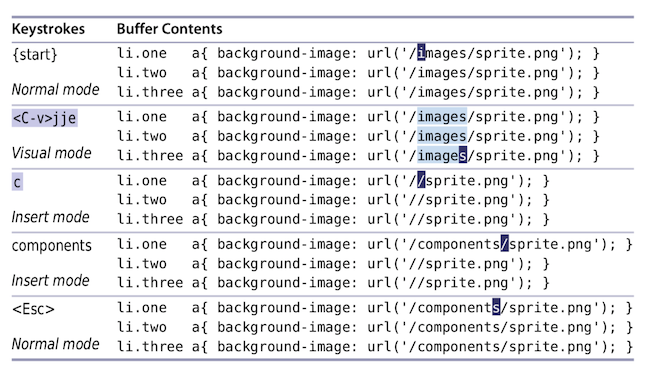Practical.Vim.2nd.Edition 实用技巧进阶: 第4章-可视化模式

# 第4章 可视模式

Visual Mode

## Tip 20 Grok可视化模式

Grok Visual Mode

`Grok` : 通过感觉意会

## Tip 21 定义视觉选择

Define a Visual Selection

Command Effect
`v` 切换为可视化模式:以字符为单位
`V` 切换为可视化模式:以行为单位
`<C-v>` 切换为可视化模式:以块为单位
`gv` 切换为可视化模式:上次的重启开启
`<Esc>` / `C-[` 切换为常规模式
`o` 可视化模式下:跳转到高亮文本的另一头

## Tip 22 重复行可视化模式命令

Repeat Line-wise Visual Commands

`:set shiftwidth=4 softtabstop=4 expandtab`

```def fib(n):
a, b = 0, 1
while a < n:
print a,
a, b = b, a+b
fib(42)
```

Keystrokes Buffer Contents
{start} def fib(n):
a, b = 0, 1
while a < n:
`p`rint a,
a, b = b, a+b
fib(42)
`Vj` def fib(n):
a, b = 0, 1
while a < n:
`print a,`
`a, b = b, a+b`
fib(42)
`>.` def fib(n):
a, b = 0, 1
while a < n:
`p`rint a,
a, b = b, a+b
fib(42)

## Tip 23 尽量优先可视命令操作符

Prefer Operators to Visual Commands Where Possible

``````<a href="#">one</a>
<a href="#">two</a>
<a href="#">three</a>
``````
Keystrokes Buffer Contents
{start} `<`a href="#">one</a>
<a href="#">two</a>
<a href="#">three</a>
`vit` <a href="#">`one`</a>
<a href="#">two</a>
<a href="#">three</a>
`U` <a href="#">`O`NE</a>
<a href="#">two</a>
<a href="#">three</a>
`j.` <a href="#">ONE</a>
<a href="#">`T`WO</a>
<a href="#">three</a>
`j.` <a href="#">ONE</a>
<a href="#">TWO</a>
<a href="#">`T`HRee</a>

`it` 表示标签内的所有内容 (`:h it`); 可视化模式下的 `U` 表示转大写 (`:h v_U`)

## Tip 24 可视化模式编辑表格

Edit Tabular Data with Visual-Block Mode

```Chapter            Page
Normal mode          15
Insert mode          31
Visual mode          44
```• `<C-v>{motion}` - Ctrl + v 开启可视化块编辑模式; 然后选择目标块
• `x...` - x删除竖向的一列空格; 后面3个 `.` 删除后续的3列空格
• `gv` - 重新选中我们最后一次的可视化模式区域
• `r|` - 当前字符替换为竖线
• `yyp` - 复制一行
• `Vr-` - 选择当前行, 字符替换为 “-“

## Tip 25 纵向编辑

Change Columns of Text

```.one     a{ background-image: url('/images/sprite.png');  }
li.two   a{ background-image: url('/images/sprite.png');  }
li.three a{ background-image: url('/images/sprite.png');  }
```## Tip 26 可视化模式追加数据

Append After a Ragged Visual Block

```var foo = 1
var bar = 'a'
var foobar = foo + bar
```
Keystrokes Buffer Contents
{start}

Normal mode
var foo = `1`
var bar = ‘a’
var foobar = foo + bar
`<C-v>jj\$`

Visual-Block
var foo = `1`
var bar = `'a'`
var foobar` = foo + bar`` `
`A;`

Insert model
var foo = 1;` `
var bar = ‘a’
var foobar = foo + bar
`<Esc>`

Normal mode
var foo = `1`;
var bar = ‘a’;
var foobar = foo + bar;

Ctrl + v 可视化块模式 下:

• `I` - 插入一段字符
• `A` - 追加字符 (每行结尾长度不一致时, 通常先使用 `\$` 定位到行尾)
• `r` - 替换为单个字符
• `c` / `s` - 替换为一段字符
• `x` - 删除字符